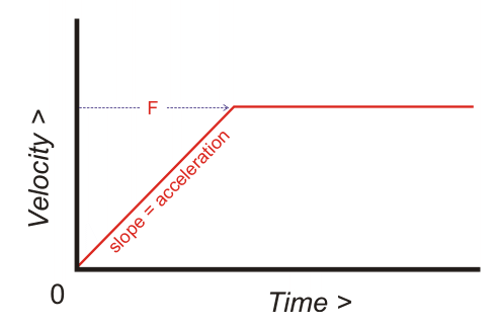Think & Tinker, Ltd.Best Acceleration to Use When Cutting With Microtools

 Acceleration is defined as the rate that a velocity changes. The acceleration that we are most concerned with is how fast an XY movement goes from 0 to the selected feedrate (F) once the controller tells the motors to start moving. In the graph below, the acceleration (also known as ΔV/ΔT) is the slope of the ramp of the red velocity plot. The steeper the ramp (higher acceleration), the quicker the axes get up to speed. The shallower the ramp (low acceleration), the longer it takes to get to "F" (F is the G-code command that sets the feedrate).From the point of view of higher performance, higher acceleration means shorter cycle times and snappier customer demos. Unfortunately, as acceleration is increased, more transient transverse stress is exerted on the bit. If the stress exceeds a certain threshold (transverse rupture point), the bit will break. This is like whacking something brittle with a hammer (or your boss's head with a new idea). A little tap might not be a big deal, but a resounding smack will send shrapnel flying. In the case of cutting soft media with small diameter carbide tools, it turns out that there is a pretty good compromise between higher performance and longer tool life. 20 inches per second per second, or 0.51m per second per second At this acceleration, your bit will think that you are coddling it like a wee baby and not break as soon as it starts to move. This is especially important as the bit starts to get dull and cutting resistance increases. Of course, if you set the feedrate (F) too high, the rupture point will eventually be exceeded and the bit will break anyway. Do not think for a minute that this acceleration is so low that your performance will suffer. At 20 in/sec/sec, you tool can get to 720 in/min in just 1 second. Axis acceleration is set using the controller software (e.g. Mach 3, EMC2, FlashCut) that was supplied with your CNC router. In the case of larger CNC machining centers, the hardware control panel (e.g. Fanuc, Fagor) is used to configure each axis. In virtually all cases, you will need to set the acceleration parameters for each axis individually. Setting Up a Rotary Axis Rotary axes present a particularly challenging case since the LINEAR acceleration of the material relative to the cutting tool will change with the distance from the rotary axis. The further out from the center of rotation you are, the higher the LINEAR acceleration is for a fixed ANGULAR acceleration. To make sure that the linear acceleration never gets too high, it is best to determine the angular acceleration necessary to result in 20 in./sec/sec (0.51m/sec/sec) at the surface of your material. The linear acceleration La on the surface of a cylinder with radius r (same units as L) rotating under the influence of an angular acceleration Φa is determined by: La = r * Φa Since La is fixed, the maximum angular acceleration is determined by: Φa(max) = La / r For example, if La = 20 in./sec/sec (0.51m/sec/sec) and r = 2.5 in. (0.0635 m), the maximum angular acceleration is determined by: Φa = (20 in./sec/sec) / 2.5 in. = 8 rad/sec/sec or for those of a metric persuasion: Φa = (0.51m/sec/sec) / 0.0635 m = 8 rad/sec/sec where "rad" is short for "radians, the dimensionless unit of angle. "Note: If your software needs angular acceleration in terms of "degrees/sec/sec", just multiply the result above by 57.3